# SSAT Middle Level Math : How to multiply variables

## Example Questions

2 Next →

### Example Question #11 : Operations

Suppose you know the values of all variables in the expression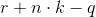and you want to evaluate the expression.

In which order will you carry out the operations?

Explanation:

By the order of operations, in the absence of grouping symbols, multiplication takes precedence over addition and subtraction. Addition and subtraction are then carried out with equal priority, but from left to right, so the addition is performed second and the subtraction last.

### Example Question #12 : Operations

Multiply in modulo 8: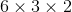None of the other choices give the correct answer.Explanation:

In modulo 8 arithmetic, a number is congruent to the remainder of the divison of that number by 8. Since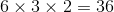and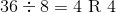then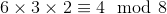.

The correct response is 4.

### Example Question #13 : Operations

Which of the following phrases can be written as the algebraic expression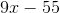?

The correct answer is not given among the other responses.

Nine multiplied by the difference of fifty-five and a number

The product of nine and a number subtracted from fifty-five

Nine multiplied by the difference of a number and fifty-five

Fifty-five subtracted from the product of nine and a number

Fifty-five subtracted from the product of nine and a number

Explanation:is fifty-five subtracted from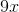.is the product of nine and a number.

Subsequently,is "fifty-five subtracted from the product of nine and a number".

### Example Question #14 : Operations

Solve the following expression,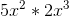.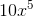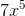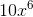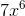Explanation:

When multiplying like variables, the constants are multiplied together.

For the exponents, when you multply variable exponents you have to add the exponents together.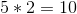and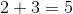so that gives you an answer of.

### Example Question #15 : Operations

Solve: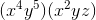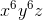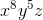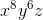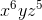Explanation:

When multiplying variables with exponents, add the exponents.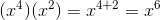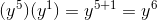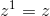The correct answer is### Example Question #16 : Operations

Solve: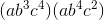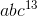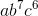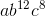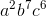Explanation:

When multiplying variables with exponents the exponents are added.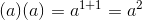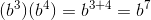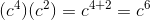The correct answer is2 Next →